Skip to content

# Tutorial: How to Plot in Owl?

Vincent Danos edited this page Mar 31, 2018 · 48 revisions

Owl is an OCaml numerical library. Besides its extensive supports to matrix operations, it also has a flexible plotting module. Owl's `Plot` module is designed to help you in making fairly complicated plots with minimal coding efforts. It is built atop of `Plplot` but hides its complexity from users.

The module is cross-platform since `Plplot` calls the underlying graphics device driver to plot. However, based on my experience, the Cairo Package provides the best quality and most accurate figure so I recommend installing Cairo.

The examples in this tutorial are generated by using `Cairo PNG Driver`.

## Create & Configure

Simply put, there are two ways of plotting: 1) with a handle; 2) without a handle. You can imagine a handle is just a "pointer" to a figure. The first option is for lazy people who do not want to fine tune their figures but want to have a look at their results quickly. Most plotting functions in Owl can be called without an explicit handle passed in. E.g., the following code creates a simple line plot.

```let f x = Maths.sin x /. x in
Plot.plot_fun f 1. 15.;;```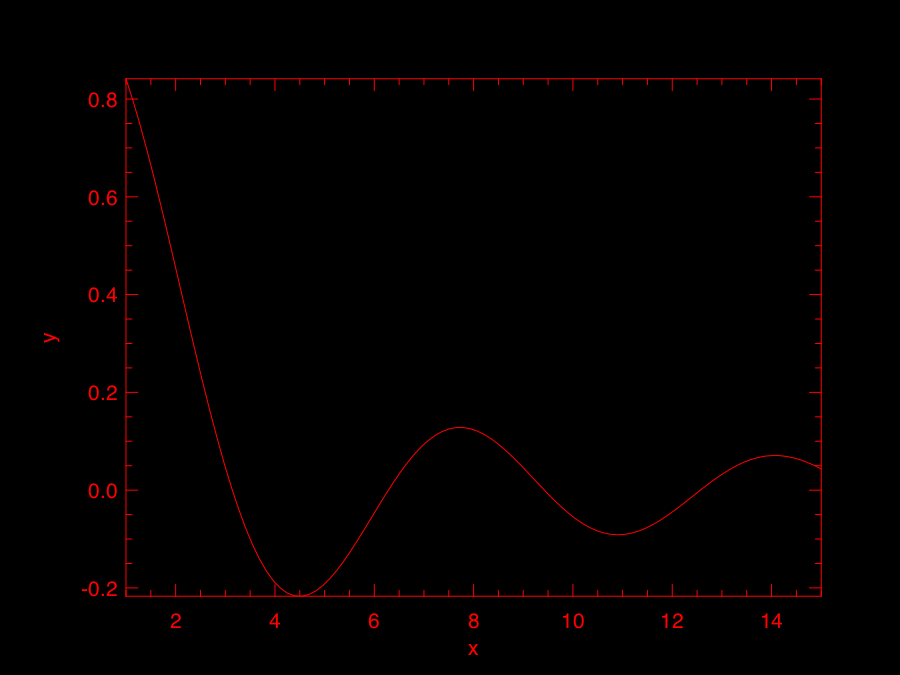However, in most cases, you do want to have a full control over the figure you are creating and do want to configure it a bit especially if you don't like the default red on black theme. Then, here is the standard way of creating a plot using `Plot.create` function. Here is its type definition in `Owl_plot.mli`

`val create : ?m:int -> ?n:int -> string -> handle`

The first two parameters `m` and `n` are used for creating subplot, I will talk about subplot later. Now we only use the third parameter which defines the output file. Let's extend the previous example.

```let f x = Maths.sin x /. x in
let h = Plot.create "plot_003.png" in
Plot.set_foreground_color h 0 0 0;
Plot.set_background_color h 255 255 255;
Plot.set_title h "Function: f(x) = sine x / x";
Plot.set_xlabel h "x-axis";
Plot.set_ylabel h "y-axis";
Plot.set_font_size h 8.;
Plot.set_pen_size h 3.;
Plot.plot_fun ~h f 1. 15.;
Plot.output h;;```

As we can see, we first create a plot handle `h` and also specified the output file as `plot_003.png` in `create` function. Owl will select the suitable output device driver for you based on the file name suffix. Then we call various functions in the `Plot` module to configure the plot by passing in `h`. These functions are self-explained, and you can read Owl's documentation for details.

There are two thing worth mentioning here. First, you need to pass `h` to the plot function, i.e., `Plot.plot_fun` in this example. Second, the most important step is calling `Plot.output h` will actually write your figure to a file.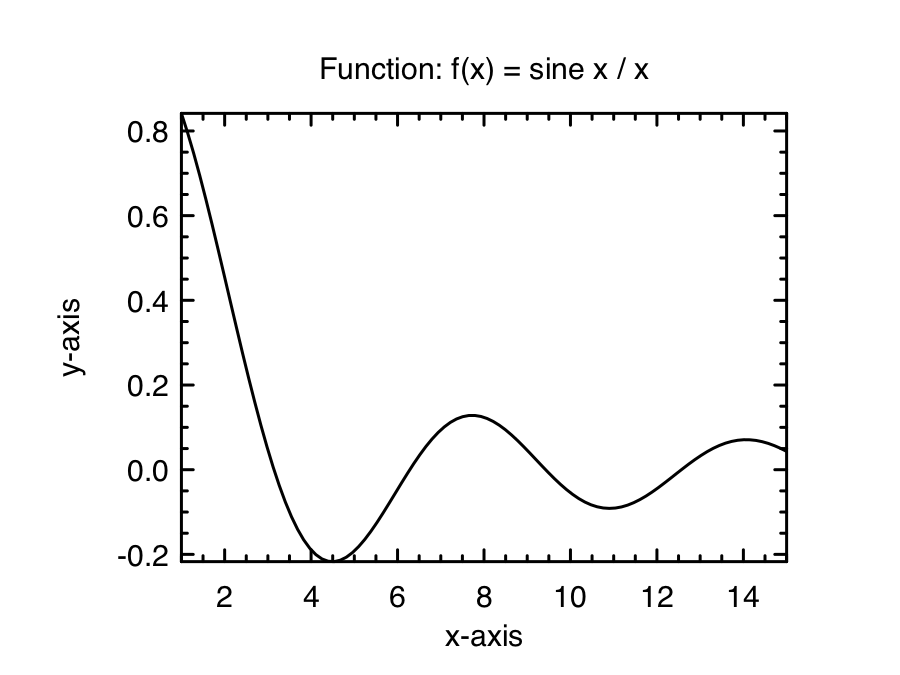Simple, isn't it? Let's continue to more interesting plots.

## Create Subplot

You use the same `Plot.create` function to start a subplot. E.g., the code below create a `2 x 2` subplot. We leave the output file name as an empty string so `Plplot` later will prompt a list of device drivers you can choose from, when you call `Plot.output`.

```let h = Plot.create ~m:2 ~n:2 "" in
...```

The key concept of subplot is: you need to successively call `Plot.subplot` to "focus" on different individual subplot to work on them. Continue the previous example, let's look at the code below.

```let f p i = match i with
| 0 -> Stats.Rnd.gaussian ~sigma:0.5 () +. p.(1)
| _ -> Stats.Rnd.gaussian ~sigma:0.1 () *. p.(0)
in
let y = Stats.gibbs_sampling f [|0.1;0.1|] 5_000 |> Mat.of_arrays in
let h = Plot.create ~m:2 ~n:2 "" in
Plot.set_background_color h 255 255 255;
(* focus on the subplot at 0,0 *)
Plot.subplot h 0 0;
Plot.set_title h "Bivariate model";
Plot.scatter ~h (Mat.col y 0) (Mat.col y 1);
(* focus on the subplot at 0,1 *)
Plot.subplot h 0 1;
Plot.set_title h "Distribution of y";
Plot.set_xlabel h "y";
Plot.set_ylabel h "Frequency";
Plot.histogram ~h ~bin:50 (Mat.col y 1);
(* focus on the subplot at 1,0 *)
Plot.subplot h 1 0;
Plot.set_title h "Distribution of x";
Plot.set_ylabel h "Frequency";
Plot.histogram ~h ~bin:50 (Mat.col y 0);
(* focus on the subplot at 1,1 *)
Plot.subplot h 1 1;
Plot.set_foreground_color h 0 50 255;
Plot.set_title h "Sine function";
Plot.(plot_fun ~h ~spec:[ LineStyle 2 ] Maths.sin 0. 28.);
Plot.autocorr ~h (Mat.sequential 1 28);
(* output your final plot *)
Plot.output h;;```

The code will generate the following plot. You can control the configuration of each individual subplot once you have "focused" on it by calling `Plot.subplot`. Besides the figure handle `h`, `Plot.subplot` uses the two-dimensional index you pass in to locate the subplot.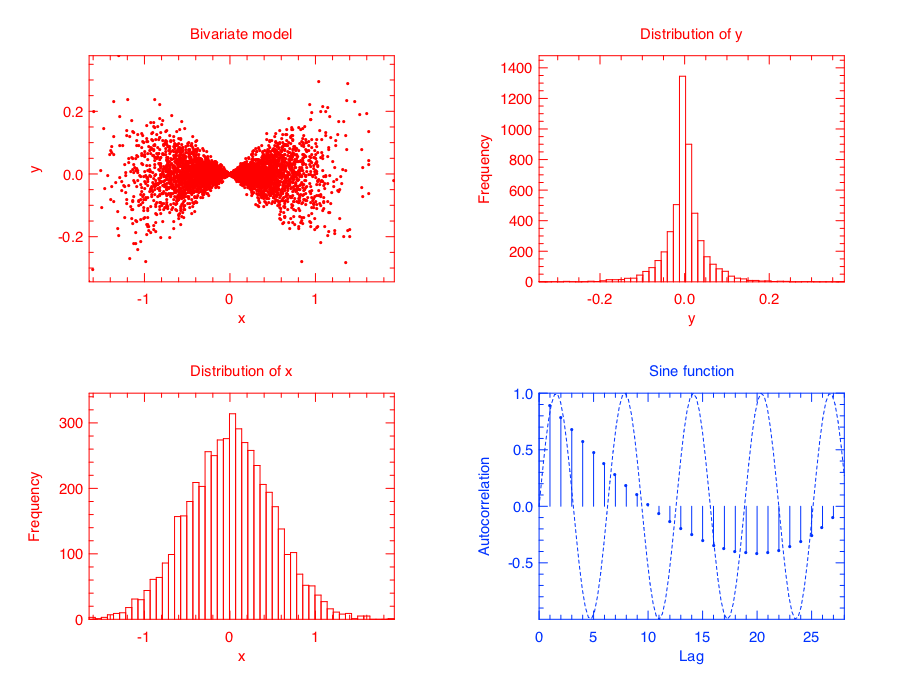`Plot` module automatically calculates the suitable page size for your subplot. If you are not happy with the calculated size, you can also specify the page size by calling `Plot.set_page_size` function.

Subplot is quite straightforward, right?

## Multiple (Lines)

You can certainly plot multiple lines (or other types of plots) on the same page. Once you call `Plot.output`, the plot will be `sealed` and written into the final file. Here is one example with both sine and cosine lines in one plot.

```let h = Plot.create "" in
Plot.(plot_fun ~h ~spec:[ RGB (0,0,255); Marker "#[0x2299]"; MarkerSize 8. ] Maths.sin 0. 9.);
Plot.(plot_fun ~h ~spec:[ RGB (255,0,0); Marker "#[0x0394]"; MarkerSize 8. ] Maths.cos 0. 9.);
Plot.legend_on h [|"Sine function"; "Cosine function"|];
Plot.output h;;```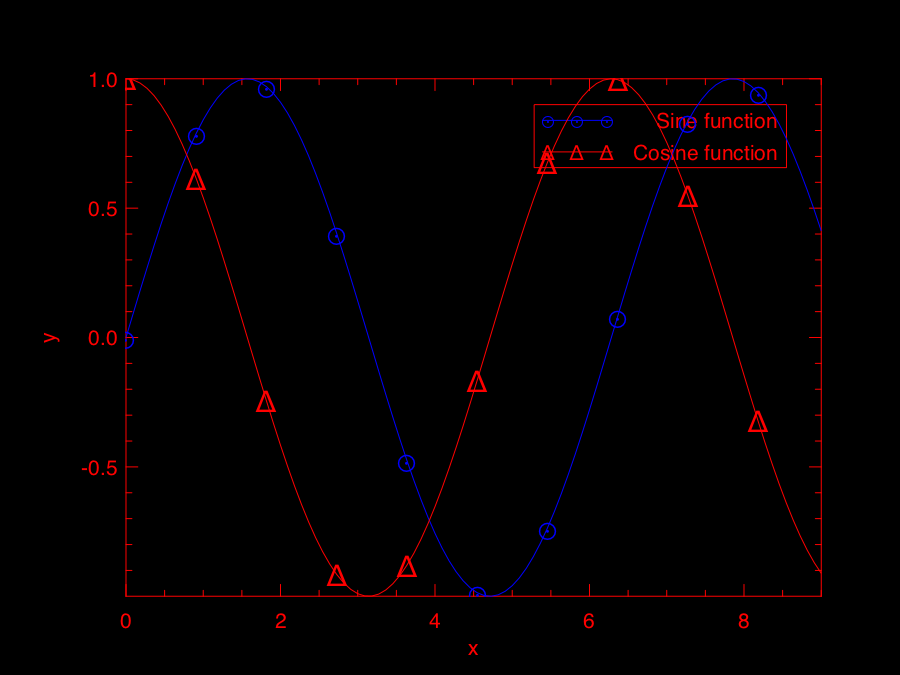Here is another example which has both histogram and line plot in one figure.

```(* generate data *)
let f p = Stats.Pdf.gaussian p.(0) 0.5 in
let g x = Stats.Pdf.gaussian x 0.5 *. 4000. in
let y = Stats.metropolis_hastings f [|0.1|] 100_000 |>  Mat.of_arrays in
(* plot multiple data sets *)
let h = Plot.create "" in
Plot.set_background_color h 255 255 255;
Plot.(histogram ~h ~spec:[ RGB (255,0,50) ] ~bin:100 y);
Plot.(plot_fun ~h ~spec:[ RGB (0,0,255); LineWidth 2. ] g (-2.) 2.);
Plot.legend_on h [|"data"; "model"|];
Plot.output h;;```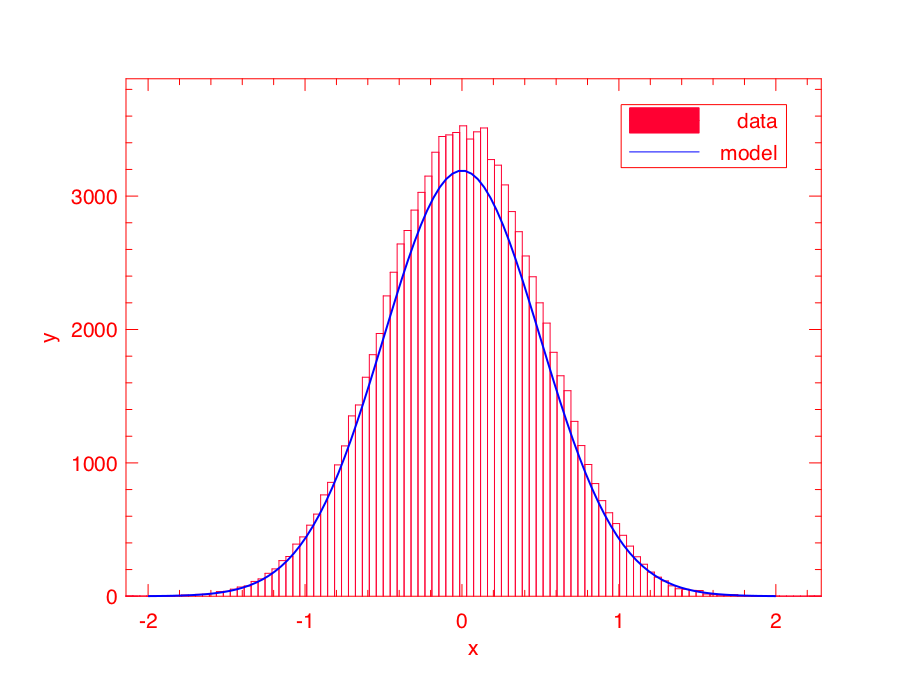So as long as you "hold" the plot without calling `Plot.output`, you can plot many data sets in one figure.

## Legend

Legend can be turned on and off by calling `Plot.legend_on` and `Plot.legend_off` respectively. When you call `Plot.legend_on`, you also need to provide an array of legend names and the position of legend. There are eight default positions in `Plot`.

```type legend_position =
North | South | West | East | NorthWest | NorthEast | SouthWest | SouthEast```

Despite of its messy looking, the following example shows how to use legend in Owl's plot module.

```(* generate data *)
let x = Mat.(uniform 1 20 *\$ 10.) in
let y = Mat.(uniform 1 20) in
let z = Mat.gaussian 1 20 in
(* plot multiple data sets *)
let h = Plot.create "" in
Plot.(plot_fun ~h ~spec:[ RGB (0,0,255); LineStyle 1; Marker "*" ] Maths.sin 1. 8.);
Plot.(plot_fun ~h ~spec:[ RGB (0,255,0); LineStyle 2; Marker "+" ] Maths.cos 1. 8.);
Plot.scatter ~h x y;
Plot.stem ~h x z;
let u = Mat.(abs(gaussian 1 10 *\$ 0.3)) in
Plot.(bar ~h ~spec:[ RGB (255,255,0); FillPattern 3 ] u);
let v = Mat.(neg u *\$ 0.3) in
let u = Mat.sequential 1 10 in
Plot.(area ~h ~spec:[ RGB (0,255,0); FillPattern 4 ] u v);
(* set up legend *)
Plot.(legend_on h ~position:NorthEast [|"test 1"; "test 2"; "scatter"; "stem"; "bar"; "area"|]);
Plot.output h;;```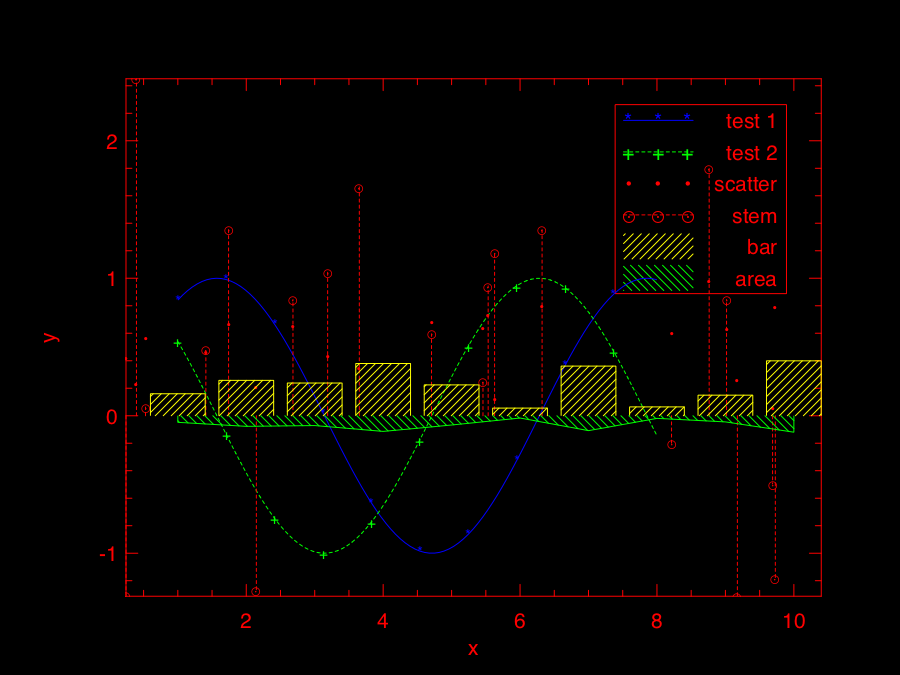## Drawing

You can draw lines and rectangles in the plot. The first example actually shows different line styles in `Plot` by drawing multiple lines.

```let h = Plot.create "" in
Plot.set_background_color h 255 255 255;
Plot.set_pen_size h 2.;
Plot.(draw_line ~h ~spec:[ LineStyle 1 ] 1. 1. 9. 1.);
Plot.(draw_line ~h ~spec:[ LineStyle 2 ] 1. 2. 9. 2.);
Plot.(draw_line ~h ~spec:[ LineStyle 3 ] 1. 3. 9. 3.);
Plot.(draw_line ~h ~spec:[ LineStyle 4 ] 1. 4. 9. 4.);
Plot.(draw_line ~h ~spec:[ LineStyle 5 ] 1. 5. 9. 5.);
Plot.(draw_line ~h ~spec:[ LineStyle 6 ] 1. 6. 9. 6.);
Plot.(draw_line ~h ~spec:[ LineStyle 7 ] 1. 7. 9. 7.);
Plot.(draw_line ~h ~spec:[ LineStyle 8 ] 1. 8. 9. 8.);
Plot.set_xrange h 0. 10.;
Plot.set_yrange h 0. 9.;
Plot.output h;;```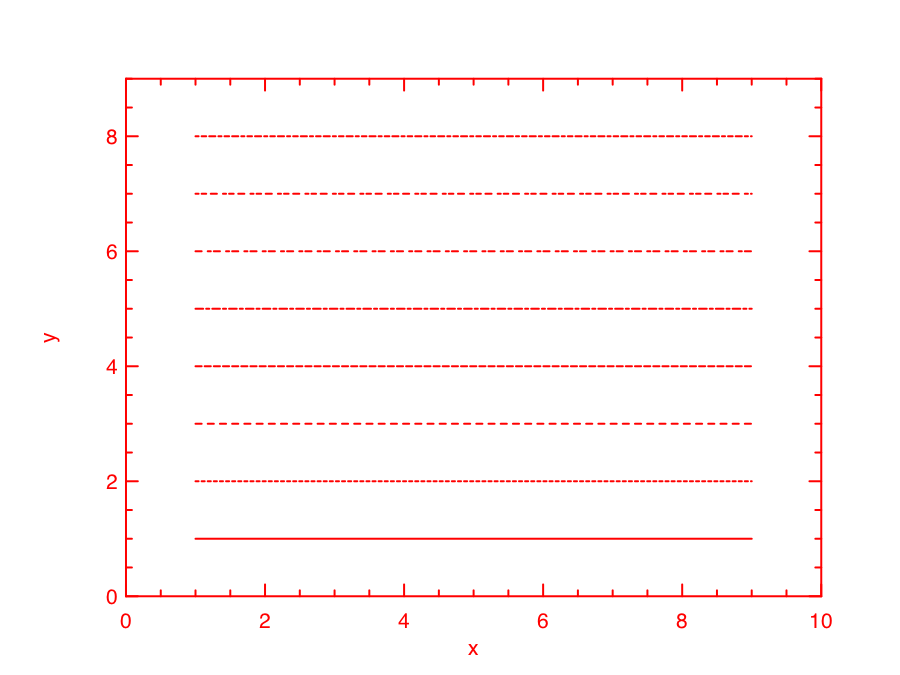Similarly, the next example shows the filling patterns in `Plot` by drawing rectangles.

```let h = Plot.create "" in
Array.init 9 (fun i ->
let x0, y0 = 0.5, float_of_int i +. 1.0 in
let x1, y1 = 4.5, float_of_int i +. 0.5 in
Plot.(draw_rect ~h ~spec:[ FillPattern i ] x0 y0 x1 y1);
Plot.(text ~h ~spec:[ RGB (0,255,0) ] 2.3 (y0-.0.2) ("pattern: " ^ (string_of_int i)));
);
Plot.output h;;```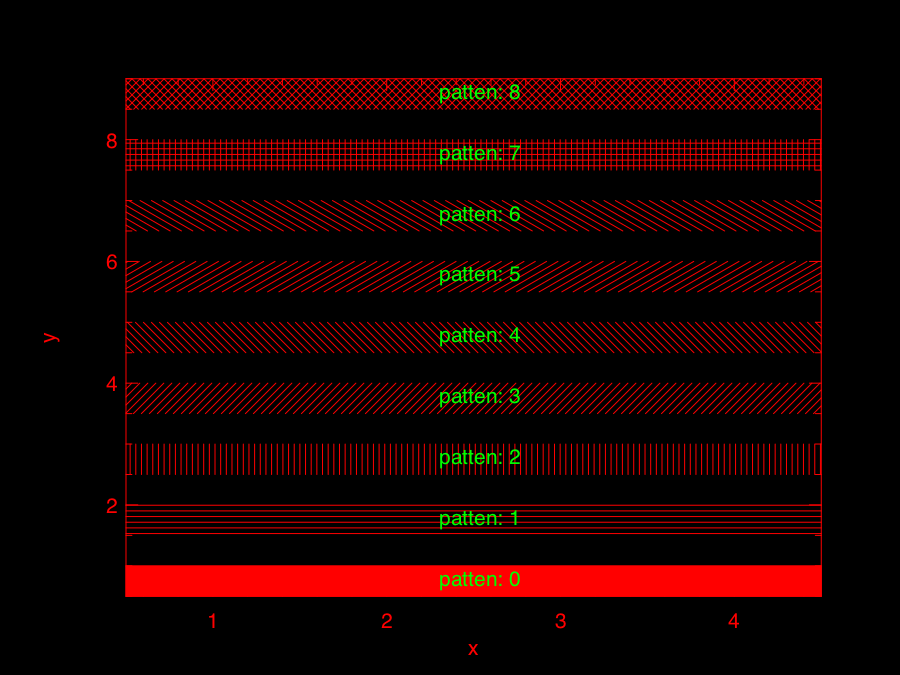## Various Plots

In the following, I will use several examples to illustrate how to use the basic plotting functions in Owl.

### Line Plot

Line plot is the most basic function. You can specify the colour, marker, and line style in the function.

```let x = Mat.linspace 0. 2. 100 in
let y0 = Mat.sigmoid x in
let y1 = Mat.map Maths.sin x in
let h = Plot.create "" in
Plot.(plot ~h ~spec:[ RGB (255,0,0); LineStyle 1; Marker "#[0x2299]"; MarkerSize 8. ] x y0);
Plot.(plot ~h ~spec:[ RGB (0,255,0); LineStyle 2; Marker "#[0x0394]"; MarkerSize 8. ] x y1);
Plot.(legend_on h ~position:SouthEast [|"sigmoid"; "sine"|]);
Plot.output h;;```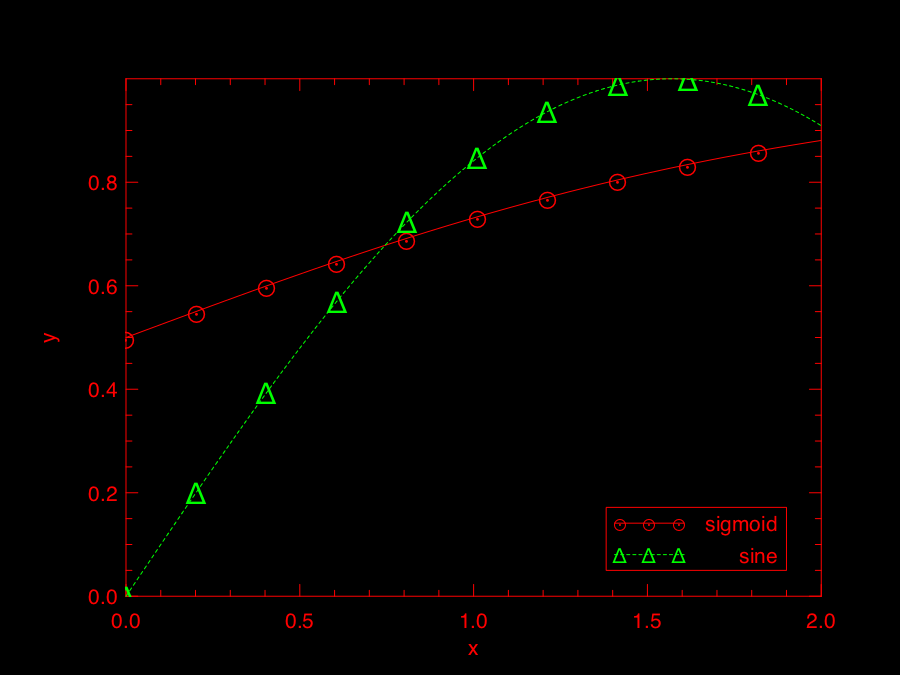### Scatter Plot

For scatter plot, the most important thing is the markers. You can check all the possible marker symbols on this page, they can be passed in as a parameter to `Plot.scatter` function.

```let x = Mat.uniform 1 30 in
let y = Mat.uniform 1 30 in
let h = Plot.create ~m:3 ~n:3 "zzz.png" in
Plot.set_background_color h 255 255 255;
Plot.subplot h 0 0;
Plot.(scatter ~h ~spec:[ Marker "#[0x2295]"; MarkerSize 5. ] x y);
Plot.subplot h 0 1;
Plot.(scatter ~h ~spec:[ Marker "#[0x229a]"; MarkerSize 5. ] x y);
Plot.subplot h 0 2;
Plot.(scatter ~h ~spec:[ Marker "#[0x2206]"; MarkerSize 5. ] x y);
Plot.subplot h 1 0;
Plot.(scatter ~h ~spec:[ Marker "#[0x229e]"; MarkerSize 5. ] x y);
Plot.subplot h 1 1;
Plot.(scatter ~h ~spec:[ Marker "#[0x2217]"; MarkerSize 5. ] x y);
Plot.subplot h 1 2;
Plot.(scatter ~h ~spec:[ Marker "#[0x2296]"; MarkerSize 5. ] x y);
Plot.subplot h 2 0;
Plot.(scatter ~h ~spec:[ Marker "#[0x2666]"; MarkerSize 5. ] x y);
Plot.subplot h 2 1;
Plot.(scatter ~h ~spec:[ Marker "#[0x22a1]"; MarkerSize 5. ] x y);
Plot.subplot h 2 2;
Plot.(scatter ~h ~spec:[ Marker "#[0x22b9]"; MarkerSize 5. ] x y);
Plot.output h;;```### Stairs Plot

Instead of drawing a straight line between two points, stairs plot first moves along the x-axis then y-axis while plotting the data. The following example is self-explained.

```let x = Mat.linspace 0. 6.5 20 in
let y = Mat.map Maths.sin x in
let h = Plot.create ~m:1 ~n:2 "" in
Plot.set_background_color h 255 255 255;
Plot.subplot h 0 0;
Plot.plot_fun ~h Maths.sin 0. 6.5;
Plot.(stairs ~h ~spec:[ RGB (0,128,255) ] x y);
Plot.subplot h 0 1;
Plot.(plot ~h ~spec:[ RGB (0,0,0) ] x y);
Plot.(stairs ~h ~spec:[ RGB (0,128,255) ] x y);
Plot.output h;;```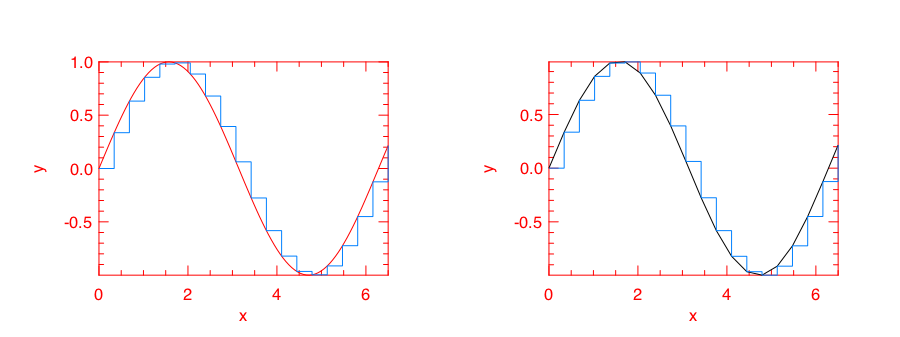### Box Plot

Box plots here generally refer to histogram, bar chart, and Whisker plot. You already learnt how to make a histogram plot. In the following, I will show how to make the other two.

Here is the example for making both bar chart and Whisker box. Just note the input to `Plot.boxplot` is a row-based matrix, each row represents multiple measurements of one variable, which correspond one box in the plot.

```let y1 = Mat.uniform 1 10 in
let y2 = Mat.uniform 10 100 in
let h = Plot.create ~m:1 ~n:2 "" in
Plot.subplot h 0 0;
Plot.(bar ~h ~spec:[ RGB (0,153,51); FillPattern 3 ] y1);
Plot.subplot h 0 1;
Plot.(boxplot ~h ~spec:[ RGB (0,153,51) ] y2);
Plot.output h;;```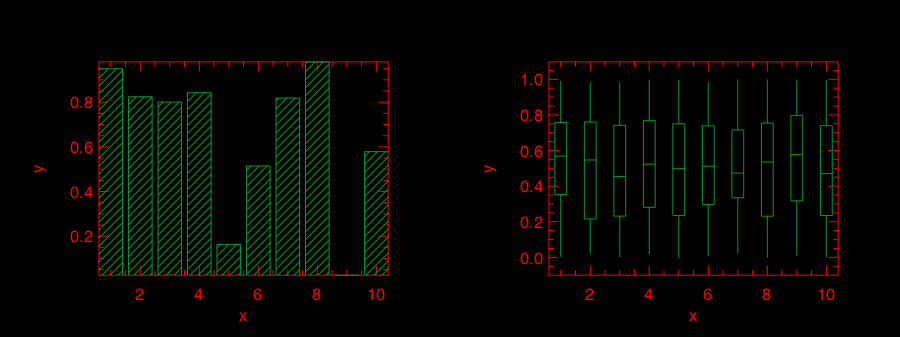### Stem Plot

Stem plot is simple, as the following code shows.

```let x = Mat.linspace 0.5 2.5 25 in
let y = Mat.map (Stats.Pdf.exponential 0.1) x in
let h = Plot.create ~m:1 ~n:2 "" in
Plot.set_background_color h 255 255 255;
Plot.subplot h 0 0;
Plot.set_foreground_color h 0 0 0;
Plot.stem ~h x y;
Plot.subplot h 0 1;
Plot.(stem ~h ~spec:[ Marker "#[0x2295]"; MarkerSize 5.; LineStyle 1 ] x y);
Plot.output h;;```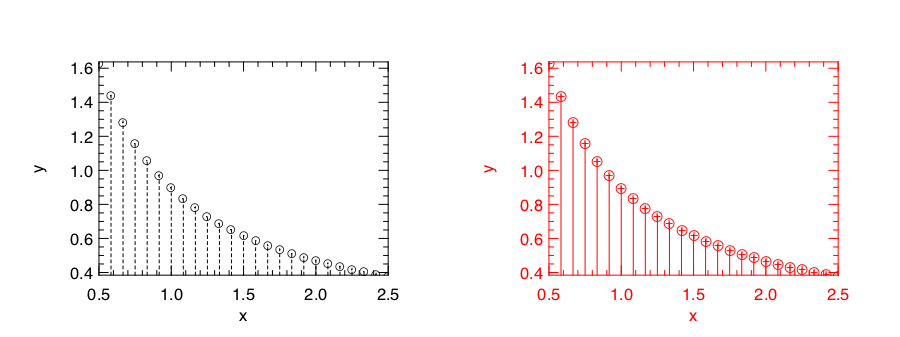Stem plot is often used to show the autocorrelation of a variable, therefore `Plot` module already includes `autocorr` for your convenience.

```let x = Mat.linspace 0. 8. 30 in
let y0 = Mat.map Maths.sin x in
let y1 = Mat.uniform 1 30 in
let h = Plot.create ~m:1 ~n:2 "" in
Plot.subplot h 0 0;
Plot.set_title h "Sine";
Plot.autocorr ~h y0;
Plot.subplot h 0 1;
Plot.set_title h "Gaussian";
Plot.autocorr ~h y1;
Plot.output h;;```

Obviously, sine function possesses stronger self-similarity than Gaussian noise.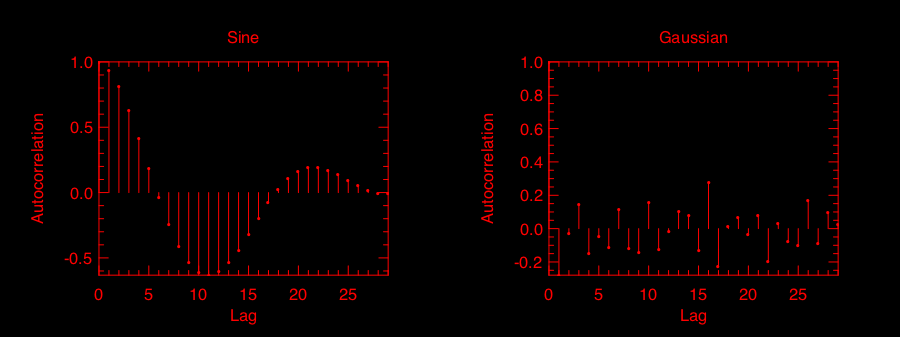### Area Plot

Area plot is similar to line plot but also fills the space between the line and x-axis.

```let h = Plot.create ~m:1 ~n:2 "" in
let x = Mat.linspace 0. 8. 100 in
let y = Mat.map Maths.atan x in
Plot.subplot h 0 0;
Plot.(area ~h ~spec:[ FillPattern 1 ] x y);
let x = Mat.linspace 0. (2. *. 3.1416) 100 in
let y = Mat.map Maths.sin x in
Plot.subplot h 0 1;
Plot.(area ~h ~spec:[ FillPattern 2 ] x y);
Plot.output h;;```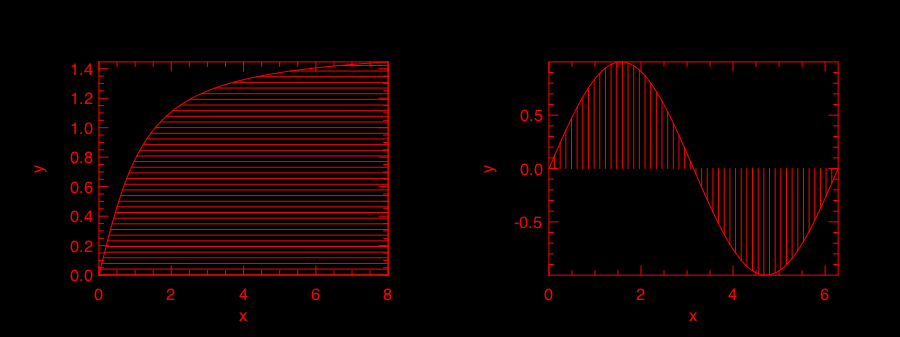### Histogram & CDF Plot

Given a series of measurements, you can easily plot the histogram and empirical cumulative distribution of the data.

```let x = Mat.gaussian 200 1 in
let h = Plot.create ~m:1 ~n:2 "" in
Plot.subplot h 0 0;
Plot.set_title h "histogram";
Plot.histogram ~h ~bin:25 x;
Plot.subplot h 0 1;
Plot.set_title h "empirical cdf";
Plot.ecdf ~h x;
Plot.output h;;```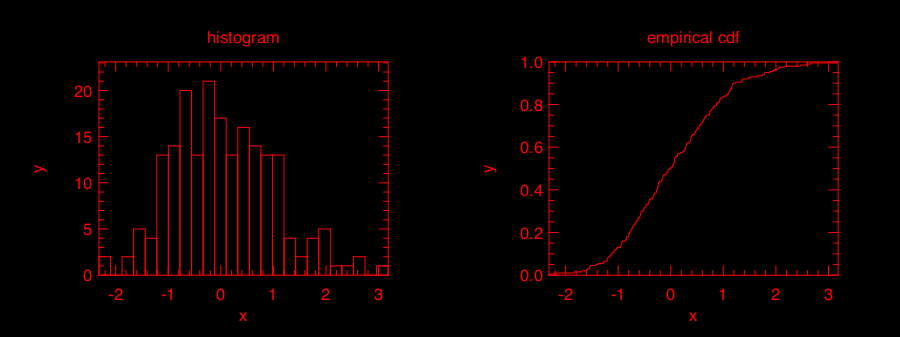### Log Plot

Plot with log-scale on either or both x and y axis.

```let x = Mat.logspace (-1.5) 2. 50 in
let y = Mat.map Maths.exp x in
let h = Plot.create ~m:2 ~n:2 "plot_027.png" in
Plot.subplot h 0 0;
Plot.set_xlabel h "Input Data X";
Plot.set_ylabel h "Input Data Y";
Plot.(loglog ~h ~spec:[ RGB (0,255,0); LineStyle 2; Marker "+" ] ~x:x y);
Plot.subplot h 0 1;
Plot.set_xlabel h "Index of Input Data Y";
Plot.set_ylabel h "Input Data Y";
Plot.(loglog ~h ~spec:[ RGB (0,0,255); LineStyle 1; Marker "*" ] y);
Plot.subplot h 1 0;
Plot.set_xlabel h "Input Data X";
Plot.set_ylabel h "Input Data Y";
Plot.semilogx ~h ~x:x y;
Plot.subplot h 1 1;
Plot.set_xlabel h "Index of Input Data Y";
Plot.set_ylabel h "Input Data Y";
Plot.semilogy ~h y;
Plot.output h;;```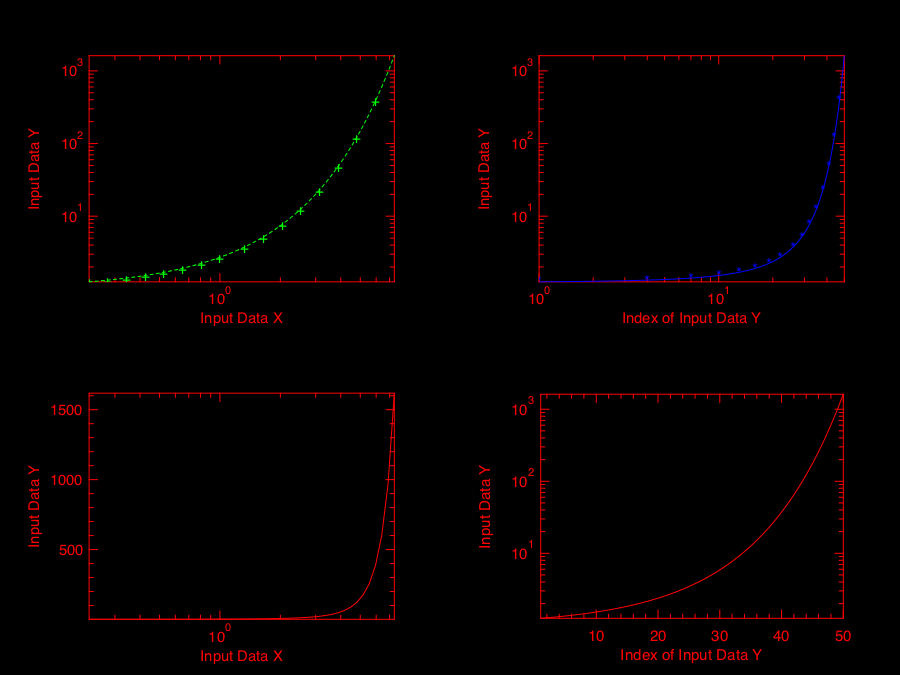### 3D Plot

There are four functions in `Plot` module related to 3D plot. They are `surf`, `mesh`, `heatmap`, and `contour` functions. Again, I will illustrate them with examples.

First, let's look at `mesh` and `surf` functions.

```let x, y = Mat.meshgrid (-2.5) 2.5 (-2.5) 2.5 100 100 in
let z0 = Mat.(sin ((x **\$ 2.) + (y **\$ 2.))) in
let z1 = Mat.(cos ((x **\$ 2.) + (y **\$ 2.))) in
let h = Plot.create ~m:2 ~n:2 "plot_016.png" in
Plot.subplot h 0 0;
Plot.surf ~h x y z0;
Plot.subplot h 0 1;
Plot.mesh ~h x y z0;
Plot.subplot h 1 0;
Plot.surf ~h x y z1;
Plot.subplot h 1 1;
Plot.mesh ~h x y z1;
Plot.output h;;```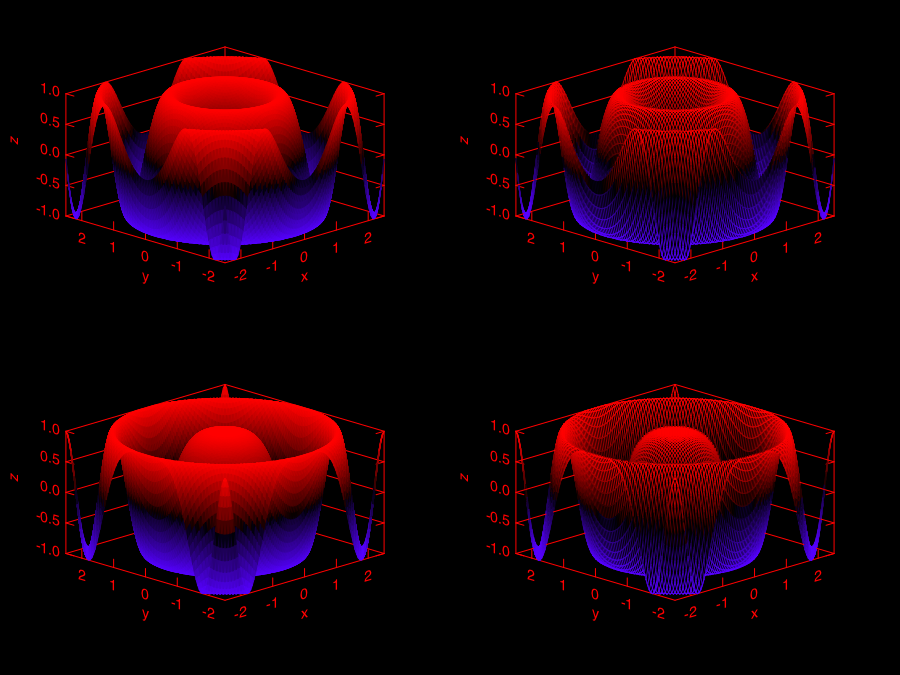It is easy to control the viewpoint with `altitude` and `azimuth` parameters. Here is an example.

```let x, y = Mat.meshgrid (-2.5) 2.5 (-2.5) 2.5 100 100 in
let z = Mat.(sin ((x * x) + (y * y))) in
let h = Plot.create ~m:1 ~n:3 "test_mesh.png" in
Plot.subplot h 0 0;
Plot.(mesh ~h ~spec:[ Altitude 50.; Azimuth 120. ] x y z);
Plot.subplot h 0 1;
Plot.(mesh ~h ~spec:[ Altitude 65.; Azimuth 120. ] x y z);
Plot.subplot h 0 2;
Plot.(mesh ~h ~spec:[ Altitude 80.; Azimuth 120. ] x y z);
Plot.output h;;```

The generated figure is as below.

image/plot_022.png

Here is another similar example with different data set.

```let x, y = Mat.meshgrid (-3.) 3. (-3.) 3. 50 50 in
let z = Mat.(
3. \$* ((1. \$- x) **\$ 2.) * exp (neg (x **\$ 2.) - ((y +\$ 1.) **\$ 2.)) -
(10. \$* (x /\$ 5. - (x **\$ 3.) - (y **\$ 5.)) * (exp (neg (x **\$ 2.) - (y **\$ 2.)))) -
((1./.3.) \$* exp (neg ((x +\$ 1.) **\$ 2.) - (y **\$ 2.)))
)
in
let h = Plot.create ~m:2 ~n:3 "plot_017.png" in
Plot.subplot h 0 0;
Plot.surf ~h x y z;
Plot.subplot h 0 1;
Plot.mesh ~h x y z;
Plot.subplot h 0 2;
Plot.(surf ~h ~spec:[ Contour ] x y z);
Plot.subplot h 1 0;
Plot.(mesh ~h ~spec:[ Contour; Azimuth 115.; NoMagColor ] x y z);
Plot.subplot h 1 1;
Plot.(mesh ~h ~spec:[ Azimuth 115.; ZLine X; NoMagColor; RGB (61,129,255) ] x y z);
Plot.subplot h 1 2;
Plot.(mesh ~h ~spec:[ Azimuth 115.; ZLine Y; NoMagColor; RGB (130,255,40) ] x y z);
Plot.output h;;```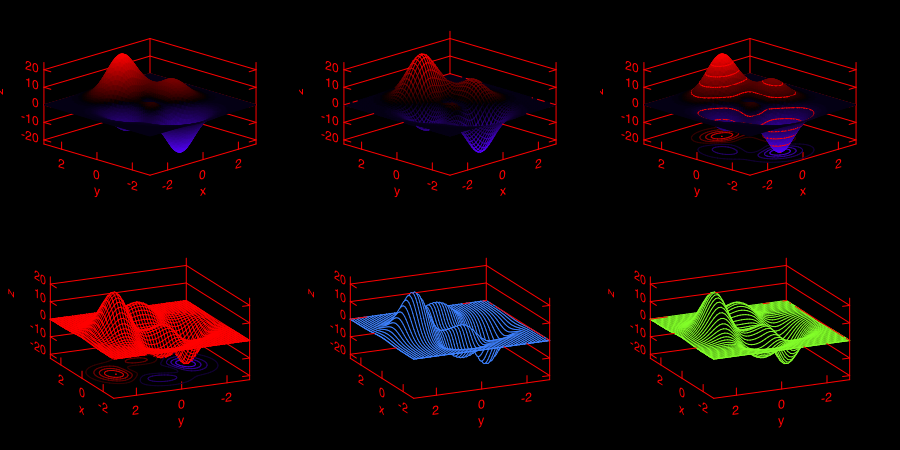Finally, let's look at how heatmap and contour look like.

```let x, y = Mat.meshgrid (-3.) 3. (-3.) 3. 100 100 in
let z = Mat.(
3. \$* ((1. \$- x) **\$ 2.) * exp (neg (x **\$ 2.) - ((y +\$ 1.) **\$ 2.)) -
(10. \$* (x /\$ 5. - (x **\$ 3.) - (y **\$ 5.)) * (exp (neg (x **\$ 2.) - (y **\$ 2.)))) -
((1./.3.) \$* exp (neg ((x +\$ 1.) **\$ 2.) - (y **\$ 2.)))
)
in
let h = Plot.create ~m:2 ~n:2 "plot_018.png" in
Plot.subplot h 0 0;
Plot.(mesh ~h ~spec:[ Contour ] x y z);
Plot.subplot h 0 1;
Plot.heatmap ~h x y z;
Plot.subplot h 1 0;
Plot.mesh ~h x y z;
Plot.subplot h 1 1;
Plot.contour ~h x y z;
Plot.output h;;```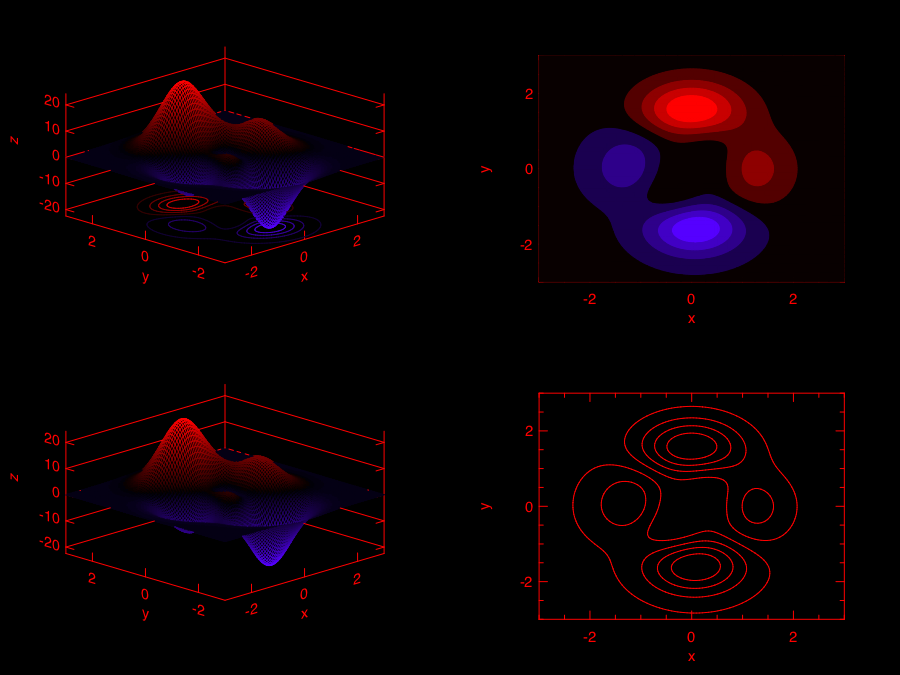### Advanced Statistical Plot

Both `qqplot` and `probplot` are simple graphical tests for determining if a data set comes from a certain distribution.

A `qqplot` displays a quantile-quantile plot of the quantiles of the sample data y versus the theoretical quantiles values from a given distribution, or the quantiles of the sample data x. Here is an example.

```let y = Mat.(gaussian 100 1 *\$ 10.) in
let x = Mat.gaussian 200 1 in
let h = Plot.create ~m:2 ~n:2 "plot_025.png" in

Plot.subplot h 0 0;
Plot.set_title h "Gaussian vs. Gaussian Sample";
Plot.set_ylabel h "Quantiles of Input Sample";
Plot.set_xlabel h "Normal Distribution Quantiles";
Plot.qqplot ~h y ~x:x;

Plot.subplot h 0 1;
Plot.set_title h "Gaussian vs. Default Dist";
Plot.set_ylabel h "Quantiles of Input Sample";
Plot.set_xlabel h "Normal Distribution Quantiles";
Plot.(qqplot ~h y ~spec:[RGB (0,128,255)]);

Plot.subplot h 1 0;
Plot.set_title h "Gaussian vs. Rayleigh Dist";
Plot.set_ylabel h "Quantiles of Input Sample";
Plot.set_xlabel h "Rayleigh Distribution (sigma=0.5) Quantiles";
Plot.qqplot ~h y ~pd:(fun p -> Stats.Cdf.rayleigh_Pinv p 0.5);

Plot.subplot h 1 1;
Plot.set_title h "Gaussian vs. Chi-Square Dist";
Plot.set_ylabel h "Quantiles of Input Sample";
Plot.set_xlabel h "Chi-Square Distribution (k=10) Quantiles";
Plot.qqplot ~h y ~pd:(fun p ->  Stats.Cdf.chisq_Pinv p 10.);

Plot.output h;;```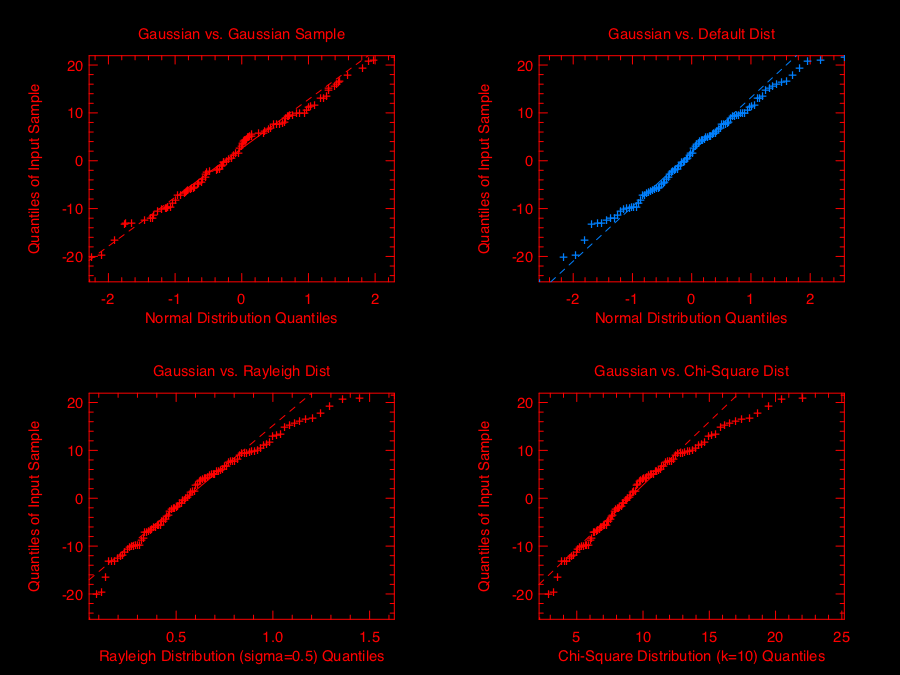`probplot` is similar to `qqplot`. It contains two special cases: `normplot` for when the given theoretical distribution is Normal distribution, and `wblplot` for Weibull Distribution. Here is an example of them.

```let x = Mat.empty 200 1 |> Mat.map (fun _ -> Stats.Rnd.weibull 1.2 1.5) in
let h = Plot.create ~m:1 ~n:2 "plot_026.png" in

Plot.subplot h 0 0;
Plot.set_title h "Random Weibull Sample vs. Std Normal Dist";
Plot.set_xlabel h "Sample Data";
Plot.set_ylabel h "Theoratical Normal Dist";
Plot.normplot ~h x;

Plot.subplot h 0 1;
Plot.set_title h "Random Weibull Sample vs. Weibull Dist";
Plot.set_xlabel h "Sample Data";
Plot.set_ylabel h "Theoratical Weibull Dist";
Plot.wblplot ~h ~lambda:1.2 ~k:1.5 x;

Plot.output h;;```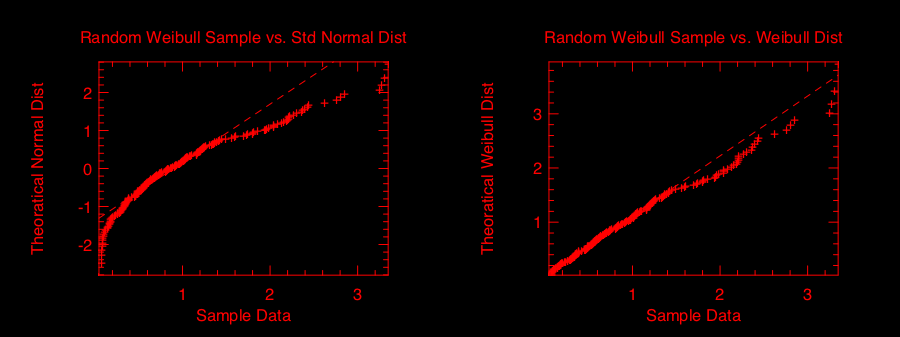### Plot Specification

For most high-level plotting functions in Owl, there is an optional parameter called `spec`. `spec` parameter take a list of specifications to let you finer control the appearance of the plot. Every function has a set of slightly different parameters, in case you pass in some parameters that a function cannot understand, they will be simply ignored. If you pass in the same parameter multiple times, only the last one will take effects.

In the following, I will provide some examples to show how to use `spec` parameter to finer tune Owl's plots. The first example shows how to configure the `mesh` plot using `ZLine`, `Contour`, and other `spec` parameters.

```let x, y = Mat.meshgrid (-2.5) 2.5 (-2.5) 2.5 50 50 in
let z = Mat.(sin ((x * x) + (y * y))) in
let h = Plot.create ~m:2 ~n:3 "plot_023.png" in

Plot.subplot h 0 0;
Plot.(mesh ~h ~spec:[ ZLine XY ] x y z);

Plot.subplot h 0 1;
Plot.(mesh ~h ~spec:[ ZLine X ] x y z);

Plot.subplot h 0 2;
Plot.(mesh ~h ~spec:[ ZLine Y ] x y z);

Plot.subplot h 1 0;
Plot.(mesh ~h ~spec:[ ZLine Y; NoMagColor ] x y z);

Plot.subplot h 1 1;
Plot.(mesh ~h ~spec:[ ZLine Y; Contour ] x y z);

Plot.subplot h 1 2;
Plot.(mesh ~h ~spec:[ ZLine XY; Curtain ] x y z);

Plot.output h;;```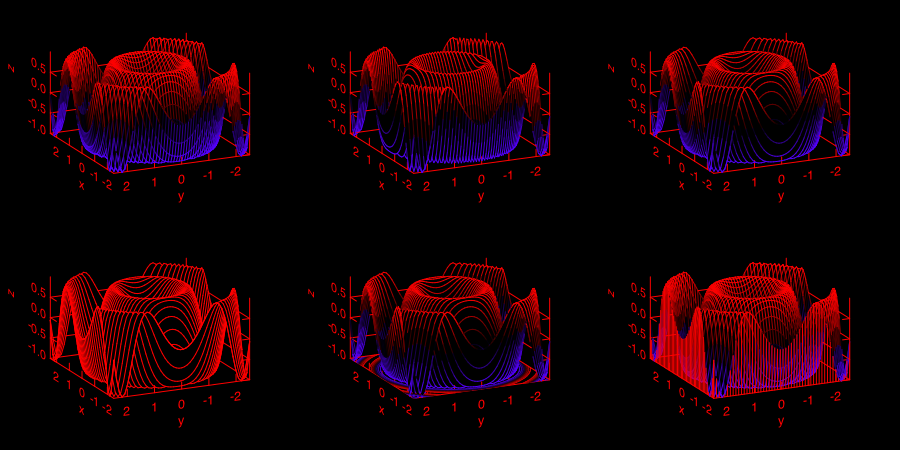The second example shows how to tune `surf` plotting function.

```let x, y = Mat.meshgrid (-1.) 1. (-1.) 1. 50 50 in
let z = Mat.(tanh ((x * x) + (y * y))) in
let h = Plot.create ~m:2 ~n:3 "plot_024.png" in

Plot.subplot h 0 0;
Plot.(surf ~h ~spec:[ ] x y z);

Plot.subplot h 0 1;
Plot.(surf ~h ~spec:[ Faceted ] x y z);

Plot.subplot h 0 2;
Plot.(surf ~h ~spec:[ NoMagColor ] x y z);

Plot.subplot h 1 0;
Plot.(surf ~h ~spec:[ Contour ] x y z);

Plot.subplot h 1 1;
Plot.(surf ~h ~spec:[ Curtain ] x y z);

Plot.subplot h 1 2;
Plot.(surf ~h ~spec:[ Altitude 10.; Azimuth 125. ] x y z);

Plot.output h;;```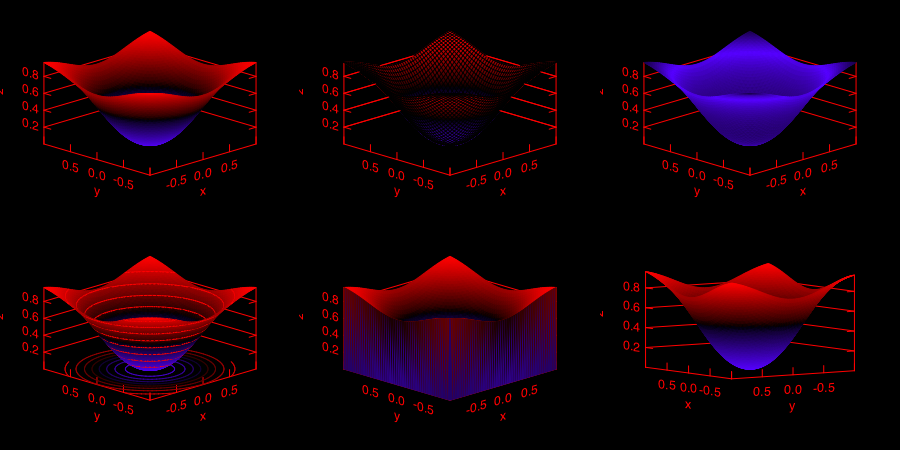## Contribute

If you have made some cool figures using Owl, please do share them with us on Plot Gallery! Thanks.

##### Clone this wiki locally
You can’t perform that action at this time.